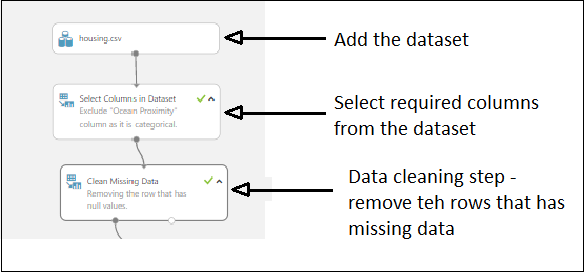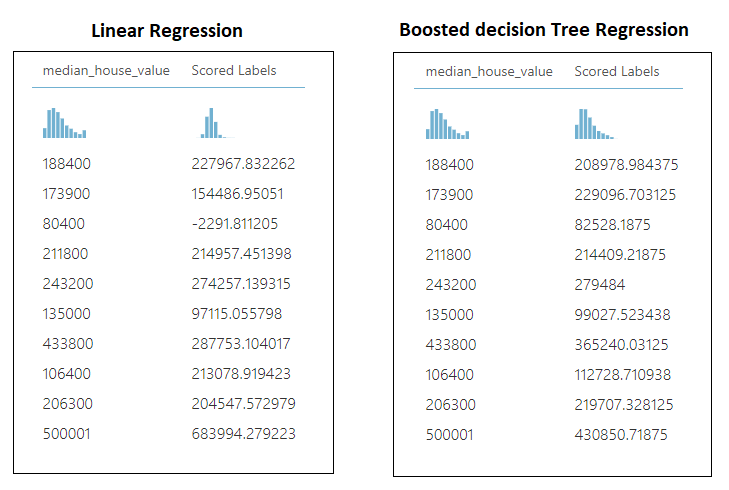top of pageSearch

# How to use Azure ML Studio to evaluate Regression Models? ML Regression model using Azure ML StudioAzure Machine Learning Studio is a Web-based integrated development environment(IDE) for building and operationalizing Machine Learning models/workflow on Azure.

I wanted to explore Azure ML Studio to build machine learning models, which I had already built using Scikit learn library in Python. The Python project code can be found here on Github.

As a beginner, wanted to build simple regression models using the California housing prices dataset from Kaggle and evaluate the outcomes. In this blog, I will be using Azure ML Studio(free version) to build ML models and evaluate them.

## Step 1: Let’s look at the dataset(Explore the dataset)

The data pertains to the houses found in a given California district and some summary stats about them based on the 1990 census data. The data is almost clean with minimal cleaning of missing data and removal of unwanted details.

The following are the columns of the dataset as described in Kaggle:

1. longitude: A measure of how far west a house is; a higher value is farther west

2. latitude: A measure of how far north a house is; a higher value is farther north

3. housingMedianAge: Median age of a house within a block; a lower number is a newer building

4. totalRooms: Total number of rooms within a block

5. totalBedrooms: Total number of bedrooms within a block

6. population: Total number of people residing within a block

7. households: Total number of households, a group of people residing within a home unit, for a block

8. medianIncome: Median income for households within a block of houses (measured in tens of thousands of US Dollars)

9. medianHouseValue: Median house value for households within a block (measured in US Dollars)

10. oceanProximity: Location of the house w.r.t ocean/sea

After exploring the dataset, medianHouseValue column is the target predicted value. Since the prediction is a single value, this is clearly a regression problem. So, I will use Linear Regression and Boosted Decision Tree Regression to build the models and evaluate the outcomes from both the models.

## Step 2: Data Wrangling

The dataset is not that clean. There are some missing values in few columns. I decided to drop the rows with missing values as they were very few.

Since oceanProximity column contains text data, I excluded that column for building the models.

In the Azure ML Studio, created a new experiment and uploaded the housing.csv that was provided by Kaggle as the dataset in the ML Studio.In the experiment canvas, add all the required modules to read data from the dataset and do data cleaning as follows:Data Preprocessing steps in ML studio experiment

## Step 3: Training the model

After the data preprocessing step, I split the dataset and used the training set to train 2 models and evaluated them as shown below:Ml studio experiment using California housing dataset

The Azure ML Studio project is available here in the Azure gallery.

The models that have been trained here along with their metrics are given below:Metrics of both the trained models

To summarize the accuracy of prediction of both the models:Azure ML Studio project scores

The same project was done in Kaggle notebook using Scikit Learn library in Python and the scores were as below:Python project scores

The Python project can be found here on my Github account.

## Step 4: Making actual predictions

The final winner is the “Boosted Decision Tree Regression” algorithm on Azure ML Studio. Both the models “Linear Regression” and the “Decision Tree Regression” algorithm on Python gave similar results.

I used a random 10 records from the dataset as the validation set to validate both the models in Azure ML Studio to check the predictions. The scored labels I got for those 10 records are shown below:Scored labels of both the regression models

We can see clearly that the predictions of “Boosted Decision Tree Regression” are fairly close to the actuals. So, we can conclude that “Boosted Decision Tree Regression” is the best model out of the 2 models we trained with the California housing dataset.

Happy Modelling!